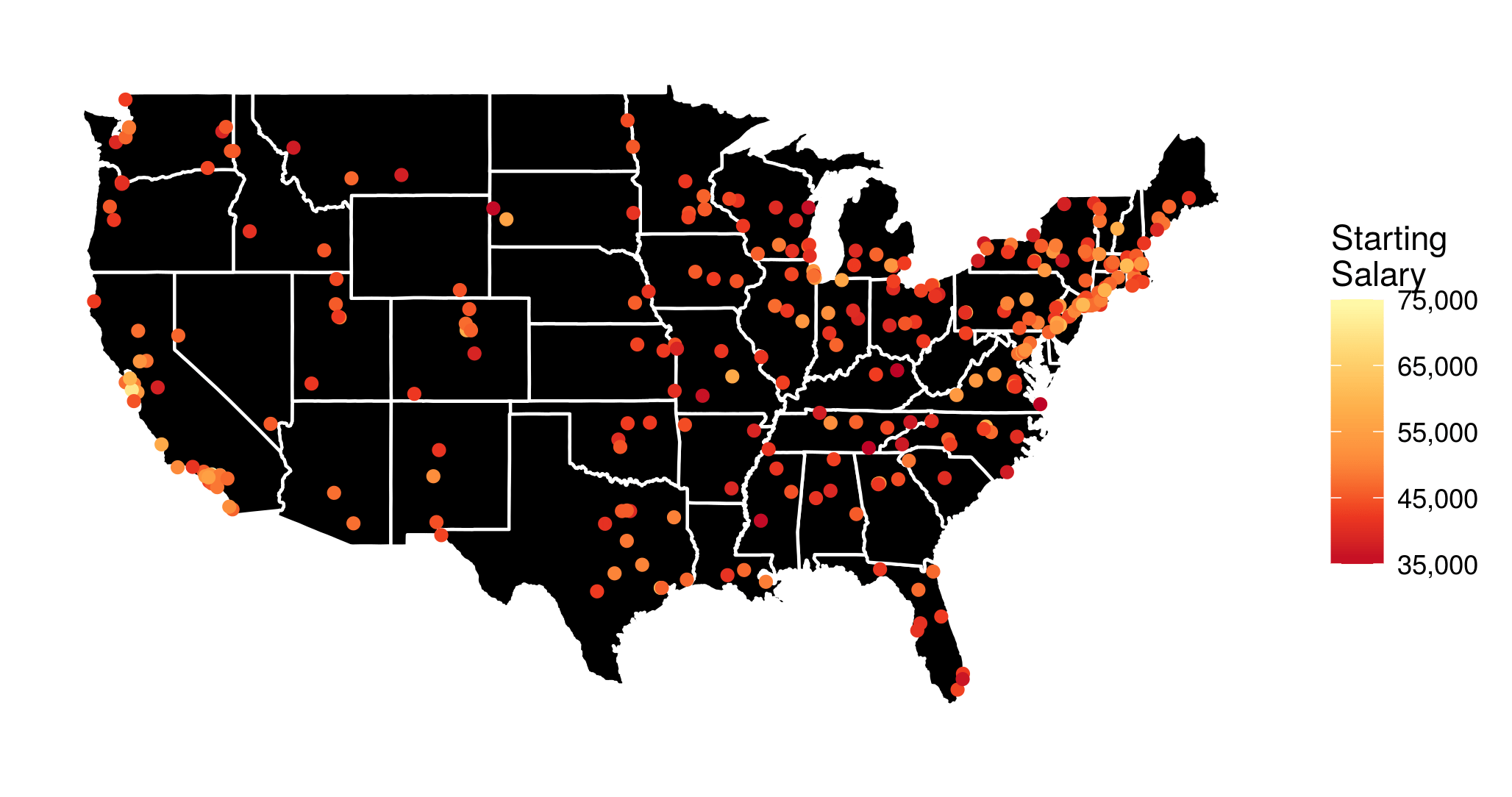Want to share your content on R-bloggers? click here if you have a blog, or here if you don't.Based on an interesting dataset from the Wall Street Journal I made the above visualization of the median starting salary for US college graduates from different undergraduate institutions (I have also looked at the mid-career salaries, and the salary increase, but more on that later). However, I thought that it would be a lot more informative, if it were interactive. To the very least I wanted to be able to see the school names when hovering over or clicking on the points with the mouse.

Luckily, this kind of interactivity can be easily achieved in R with the library `plotly`, especially due to its excellent integration with `ggplot2`, which I used to produce the above figure. In the following I describe how exactly this can be done.

Before I show you the interactive visualizations, a few words on the data preprocessing, and on how the map and the points are plotted with `ggplot2`:

• I generally use functions from the tidyverse R packages.
• I save the data in the data frame `salaries`, and transform the given amounts to proper floating point numbers, stripping the dollar signs and extra whitespaces.
• The data provide school names. However, I need to find out the exact geographical coordinates of each school to put it on the map. This can be done in a very convenient way, by using the `geocode` function from the `ggmap` R package:
```school_longlat <- geocode(salaries\$school)
school_longlat\$school <- salaries\$school
salaries <- left_join(salaries, school_longlat)
```
• For the visualization I want to disregard the colleges in Alaska and Hawaii to avoid shrinking the rest of the map. The respective rows of `salaries` can be easily determined with a `grep` search:
```grep("alaska", salaries\$school, ignore.case = 1)
#  206
grep("hawaii", salaries\$school, ignore.case = 1)
#  226
```
• A data frame containing geographical data that can be used to plot the outline of all US states can be loaded using the function `map_data` from the `ggplot2` package:
```states <- map_data("state")
```
• And I load a yellow-orange-red palette with the function `brewer.pal` from the `RColorBrewer` library, to use as a scale for the salary amounts:
```yor_col <- brewer.pal(6, "YlOrRd")
```
• Finally the (yet non-interactive) visualization is created with `ggplot2`:
```p <- ggplot(salaries[-c(206, 226), ]) +
geom_polygon(aes(x = long, y = lat, group = group),
data = states, fill = "black",
color = "white") +
geom_point(aes(x = lon, y = lat,
color = starting, text = school)) +
coord_fixed(1.3) +
colors = rev(yor_col),
labels = comma) +
guides(size = FALSE) +
theme_bw() +
theme(axis.text = element_blank(),
axis.line = element_blank(),
axis.ticks = element_blank(),
panel.border = element_blank(),
panel.grid = element_blank(),
axis.title = element_blank())
```

Now, entering `p` into the R console will generate the figure shown at the top of this post.

However, we want to…

## …make it interactive

The function `ggplotly` immediately generates a plotly interactive visualization from a `ggplot` object. It’s that simple! :smiley: (Though I must admit that, more often than I would be okay with, some elements of the ggplot visualization disappear or don’t look as expected. :fearful:)

The function argument `tooltip` can be used to specify which aesthetic mappings from the `ggplot` call should be shown in the tooltip. So, the code

```ggplotly(p, tooltip = c("text", "starting"),
width = 800, height = 500)
```

generates the following interactive visualization.

Now, if you want to publish a plotly visualization to https://plot.ly/, you first need to communicate your account info to the plotly R package:

```Sys.setenv("plotly_username" = "??????")
Sys.setenv("plotly_api_key" = "????????????")
```

and after that, posting the visualization to your account at https://plot.ly/ is as simple as:

```plotly_POST(filename = "Starting", sharing = "public")
```

## More visualizations

Finally, based on the same dataset I have generated an interactive visualization of the median mid-career salaries by undergraduate alma mater (the R script is almost identical to the one described above). The resulting interactive visualization is embedded below.

Additionally, it is quite informative to look at a visualization of the salary increase from starting to mid-career.